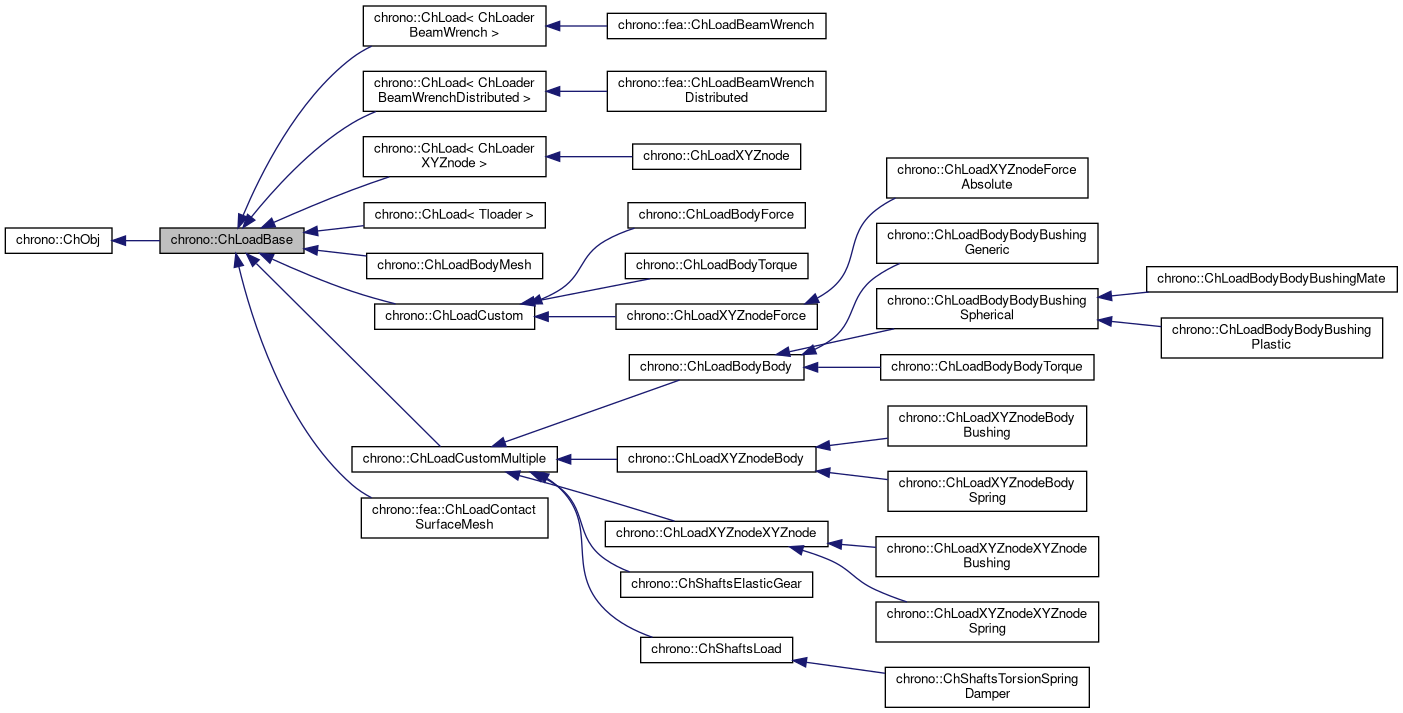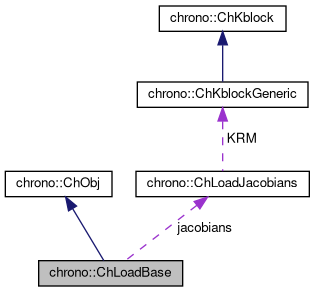## Description

This class can be inherited to implement applied loads to bodies, finite elements, etc. A load is an object that might wrap one or more ChLoader objects, whose value is dependent. It implements functionalities to perform automatic differentiation of the load so it optionally can compute the jacobian (the tangent stiffness matrix of the load) that can be used in implicit integrators, statics, etc.

#include <ChLoad.h>[legend][legend]

## Public Member Functions

Gets the number of DOFs affected by this load (position part)

Gets the number of DOFs affected by this load (speed part)

Gets all the current DOFs packed in a single vector (position part)

Gets all the current DOFs packed in a single vector (speed part)

virtual void LoadStateIncrement (const ChState &x, const ChStateDelta &dw, ChState &x_new)=0
Increment a packed state (ex. More...

Number of coordinates in the interpolated field, ex=3 for a tetrahedron finite element or a cable, = 1 for a thermal problem, etc.

virtual void ComputeQ (ChState *state_x, ChStateDelta *state_w)=0
Compute Q, the generalized load(s). More...

virtual void ComputeJacobian (ChState *state_x, ChStateDelta *state_w, ChMatrixRef mK, ChMatrixRef mR, ChMatrixRef mM)=0
Compute the K=-dQ/dx, R=-dQ/dv , M=-dQ/da jacobians. More...

Access the jacobians (if any, i.e. if this is a stiff load)

virtual void CreateJacobianMatrices ()=0
Create the jacobian loads if needed, and also set the ChVariables referenced by the sparse KRM block.

virtual void Update (double time)
Update: this is called at least at each time step. More...

virtual bool IsStiff ()=0
Report if this is load is stiff. More...

Adds the internal loads Q (pasted at global nodes offsets) into a global vector R, multiplied by a scaling factor c, as R += forces * c.

virtual void InjectKRMmatrices (ChSystemDescriptor &mdescriptor)
Tell to a system descriptor that there are item(s) of type ChKblock in this object (for further passing it to a solver) Basically does nothing, but inherited classes must specialize this.

virtual void KRMmatricesLoad (double Kfactor, double Rfactor, double Mfactor)
Adds the current stiffness K and damping R and mass M matrices in encapsulated ChKblock item(s), if any. More...Public Member Functions inherited from chrono::ChObj
ChObj (const ChObj &other)

virtual ChObjClone () const =0
"Virtual" copy constructor. More...

int GetIdentifier () const
Gets the numerical identifier of the object.

void SetIdentifier (int id)
Sets the numerical identifier of the object.

double GetChTime () const
Gets the simulation time of this object.

void SetChTime (double m_time)
Sets the simulation time of this object.

const char * GetName () const
Gets the name of the object as C Ascii null-terminated string -for reading only!

void SetName (const char myname[])
Sets the name of this object, as ascii string.

std::string GetNameString () const
Gets the name of the object as C Ascii null-terminated string.

void SetNameString (const std::string &myname)
Sets the name of this object, as std::string.

void MFlagsSetAllOFF (int &mflag)

void MFlagsSetAllON (int &mflag)

void MFlagSetON (int &mflag, int mask)

void MFlagSetOFF (int &mflag, int mask)

int MFlagGet (int &mflag, int mask)

virtual void ArchiveOUT (ChArchiveOut &marchive)
Method to allow serialization of transient data to archives.

virtual void ArchiveIN (ChArchiveIn &marchive)
Method to allow de-serialization of transient data from archives.

virtual std::string & ArchiveContainerName ()

## Protected AttributesProtected Attributes inherited from chrono::ChObj
double ChTime
the time of simulation for the object

## ◆ ComputeJacobian()

 virtual void chrono::ChLoadBase::ComputeJacobian ( ChState * state_x, ChStateDelta * state_w, ChMatrixRef mK, ChMatrixRef mR, ChMatrixRef mM )
pure virtual

Compute the K=-dQ/dx, R=-dQ/dv , M=-dQ/da jacobians.

Called automatically at each Update().

Parameters
 state_x state position to evaluate jacobians state_w state speed to evaluate jacobians mK result dQ/dx mR result dQ/dv mM result dQ/da

## ◆ ComputeQ()

 virtual void chrono::ChLoadBase::ComputeQ ( ChState * state_x, ChStateDelta * state_w )
pure virtual

Where Q is stored depends on children classes. Called automatically at each Update().

Parameters
 state_x state position to evaluate Q state_w state speed to evaluate Q

## ◆ IsStiff()

pure virtual

Report if this is load is stiff.

If so, InjectKRMmatrices will provide the jacobians of the load.

virtual

Adds the current stiffness K and damping R and mass M matrices in encapsulated ChKblock item(s), if any.

The K, R, M matrices are added with scaling values Kfactor, Rfactor, Mfactor.

 virtual void chrono::ChLoadBase::LoadStateIncrement ( const ChState & x, const ChStateDelta & dw, ChState & x_new )
pure virtual

Increment a packed state (ex.

as obtained by LoadGetStateBlock_x()) by a given packed state-delta. Compute: x_new = x + dw. Ex. this is called by the BDF numerical differentiation routine that computes jacobian in the default ComputeJacobian() fallback, if not overriding ComputeJacobian() with an analytical form.

## ◆ Update()

 void chrono::ChLoadBase::Update ( double time )
virtual

Update: this is called at least at each time step.

• It recomputes the generalized load Q vector(s)
• It recomputes the jacobian(s) K,R,M in case of stiff load Q and jacobians assumed evaluated at the current state. Jacobian structures are automatically allocated if needed.